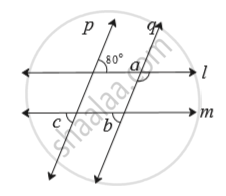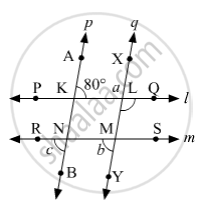Advertisement Remove all ads

# In the Adjoining Figure. Line P || Line Q. Line L || Line M. Find Measures of ∠A, ∠B, and ∠C, Using the Measures of Given Angles. Justify Your Answers. - Mathematics

Sum

In the adjoining figure. line p || line q.  line l || line m. Find measures of ∠a, ∠b, and ∠c, using the measures of given angles. Justify your answers.Advertisement Remove all ads

#### SolutionLet us mark the points A and B on p; X and Y on q; P and Q on l; R and S on m.
Suppose AB and XY intersect PQ at K and L respectively.
Suppose AB and XY intersect RS at N and M respectively.
Since, p||q and l is a transversal, then
m∠AKL + m∠XLK = 180°    (Interior angles on same side of transversal are supplementary)
⇒ 80° + m∠XLK = 180°
⇒ m∠XLK = 180° − 80°
⇒ m∠XLK = 100°
Since, PQ and XY are straight lines that intersect at L, then
m∠QLM = m∠XLK    (Vertically opposite angles)
⇒ a = 100°
Since, l||m and p is a transversal, then
m∠BNR = m∠AKL    (Alternate exterior angles)
⇒ c = 80°
Since, p||q and m is a transversal, then
m∠NMY= m∠RNB    (Corresponding angles)
⇒ b = c
⇒ b = 80°

Concept: Properties of Angles Formed by Two Parallel Lines and a Transversal
Is there an error in this question or solution?
Advertisement Remove all ads

#### APPEARS IN

Balbharati Mathematics 8th Standard Maharashtra State Board
Chapter 2 Parallel lines and transversals
Practice Set 2.2 | Q 3 | Page 12
Advertisement Remove all ads
Advertisement Remove all ads
Share
Notifications

View all notifications

Forgot password?